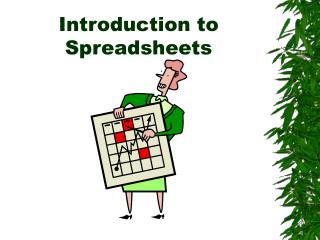# Introduction to Spreadsheets - PowerPoint PPT PresentationDownload PresentationDownload Presentation- - - - - - - - - - - - - - - - - - - - - - - - - - - E N D - - - - - - - - - - - - - - - - - - - - - - - - - - -
##### Presentation Transcript

2. What are Uses of Spreadsheets? • Prepare budgets • Maintain student grades • Prepare financial statements • Analyze numbers • Manage inventory • Make forecasts

3. Advantages of Spreadsheets • Forecasting and making decisions • Fast and efficient • Automatically recalculates

4. What-if Analysis A spreadsheet tool used to play out different situations to determine the outcome

5. Spreadsheet Terminology • Cell – the intersection of a row and a column • Active cell - The cell in which you are currently working. • Cell address - The location of a cell as identified by the column letter and row number.

6. Parts of the Spreadsheet Window Active cell cells Column C Row 5

7. Entering Data into a Spreadsheet • Labels • Alphabetical text on a spreadsheet. They tell you what the values mean. • Values • A number entered into a spreadsheet • Formulas • Statement that performs a calculation • Functions • Built-in formula that is a shortcut for common calculations

8. Identifying Labels and Values labels values

9. Identifying Formulas and Functions formula The result of the formula function The result of the function

10. Identifying Parts of a Spreadsheet Formula mathematical operators =B2+C2+D2 equal sign—first part of any spreadsheet formula cell reference/address—made up of the column heading and the row number

11. Identifying Parts of a Spreadsheet Function =SUM(B2:B6) name of function range equal sign

12. Mathematical Operators + addition - subtraction * multiplication (asterisk) / division (slash or diagonal)

13. Spreadsheet Functions Function NameDescription AVERAGE Average of arguments. COUNT Counts the number of cells in a range. IF Specifies a logical test to perform; then performs one action if test result in true and another if it is not true. MAX Maximum value of range of cells. MIN Minimum value of range of cells. ROUND Rounds a number to a specified number of digits. SUM Totals a range of cells.

14. Relative and Absolute Referencing • Relative • cells that change when they are copied into other cells • Absolute • cells that do not change regardless of where it is copied

15. Changing Column Width 1. Position cursor between column headings. 2. Make sure your cursor changes to cross-hairs. 3. Click and drag to the right. Shortcut: double-click between the column headings!

16. Changing Row Height 1. Position cursor between row headings. 2. Make sure your cursor changes to cross-hairs. 3. Click and drag down. Shortcut: double-click between the row headings!

17. Formatting Cells with Enhancements underline shading font size bold borders

18. Numeric Formatting decimal currency general

19. Print Orientations • Portrait • A paper orientation that is taller than it is wide. • Landscape • A paper orientation that is wider than it is tall.

20. Print Options without gridlines with gridlines